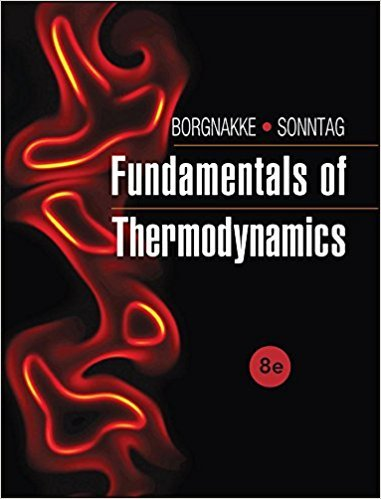×
Get Full Access to Fundamentals Of Thermodynamcs - 8 Edition - Chapter 5 - Problem 39hp
Get Full Access to Fundamentals Of Thermodynamcs - 8 Edition - Chapter 5 - Problem 39hp

×

# Discuss the factors that would make the heat pumpISBN: 9781118131992 58

## Solution for problem 39HP Chapter 5

Fundamentals of Thermodynamcs | 8th Edition

• Textbook Solutions
• 2901 Step-by-step solutions solved by professors and subject experts
• Get 24/7 help from StudySoup virtual teaching assistantsFundamentals of Thermodynamcs | 8th Edition

4 5 1 288 Reviews
21
1
Problem 39HP

Problem 39HP

Discuss the factors that would make the heat pump described in Problem 4.123 an irreversible cycle.

Problem 4.123

An R-410a heat pump cycle shown in Fig. P4.123 has an R-410a flow rate of 0.05 kg/s with 5 kW into the compressor. The following data are given:

 State 1 2 3 4 5 6 P, kPa 3100 3050 3000 420 400 390 T, °C 120 110 45 −10 −5 h, kJ/kg 377 367 134 — 280 284

Calculate the heat transfer from the compressor, the heat transfer from the R-410a in the condenser, and the heat transfer to the R-410a in the evaporator.

FIGURE P4.123Step-by-Step Solution:

Solution 39 HP:

Step 1 of 1:-

Here we have to discuss the factors that would make the heat pump as irreversible cycle.

The mass flow rate is 0.05 kg/s.

The discussion is shown below:

• The pressure will be decreased through all the lines.

• The inside pressure of the compressor will also be decreased.

• There will be catastrophic heat transfer into the compressor which will cause severe damage.

• There will be leak in the backward flow which will lead to decrease in pressure abruptly.

• In line 3 to 4, there will be throttle process.
Step 2 of 1

##### ISBN: 9781118131992

The full step-by-step solution to problem: 39HP from chapter: 5 was answered by , our top Engineering and Tech solution expert on 08/03/17, 05:05AM. Since the solution to 39HP from 5 chapter was answered, more than 279 students have viewed the full step-by-step answer. This textbook survival guide was created for the textbook: Fundamentals of Thermodynamcs , edition: 8. The answer to “Discuss the factors that would make the heat pump described in 4.123 an irreversible cycle. 4.123An R-410a heat pump cycle shown in Fig. P4.123 has an R-410a flow rate of 0.05 kg/s with 5 kW into the compressor. The following data are given:State123456P, kPa310030503000420400390T, °C12011045 ?10?5h, kJ/kg377367134—280284Calculate the heat transfer from the compressor, the heat transfer from the R-410a in the condenser, and the heat transfer to the R-410a in the evaporator.FIGURE P4.123” is broken down into a number of easy to follow steps, and 73 words. Fundamentals of Thermodynamcs was written by and is associated to the ISBN: 9781118131992. This full solution covers the following key subjects: heat, transfer, compressor, cycle, Pump. This expansive textbook survival guide covers 7 chapters, and 1462 solutions.

Unlock Textbook Solution